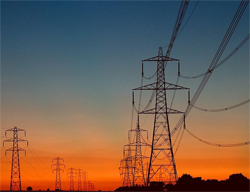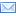#Difference Between Similar Terms and Objects

# Difference Between Energy and PowerPeople always seem to consider Energy and Power to be the same. They even make the mistake of thinking ‘Energy and Power’ as synonyms. Well, one cannot even be blamed of finding a similarity between Energy and Power as they are interrelated.

It is not that difficult to distinguish between Energy and power. While energy is the ability to do work, Power is its measurement, which calculates the time by which the energy has been used. Well, Energy is what one delivers and Power is the rate at which it is delivered.

Energy is the capability to do something. For example, energy is used for moving the car or heating the home or lighting the night or even flying an aeroplane. The basic unit of Energy is Joule but normally it is termed in watt-hour or kilowatt-hour. Energy appears in many forms and is often expressed in multiple units.

Coming to power, it is rate of Energy per unit of time. Power is the capacity of energy, which is being used. In more simple terms, power is defined as the rate of doing work. Power finds it use in mechanical applications, heat applications, electrical applications and several other areas.

Let us see an example of a weight lifter to understand Energy and Power more precisely. Power is like the strength of a weight lifter and Energy is the measure of how long he can sustain the output of power. While energy is ‘joules’, power is ‘joules per second’. Well, in another words Power is ‘watt’ and Energy is ‘watt-hour’.

Another difference is that energy can be stored whereas power cannot be stored. While energy comes with a time component, Power is an instantaneous quantity. Power cannot vary but remains constant. Meanwhile energy accumulates predictably.

Energy changes form but power doesn’t change form. If something has to happen, Energy has to change form. But Power only measures how fast the change has happened; Power is the rate at which the energy has been converted every second.Find more information related energy and power.

### Search DifferenceBetween.net :

Custom Search

Help us improve. Rate this post!(14 votes, average: 4.14 out of 5)Loading...Email This Post : If you like this article or our site. Please spread the word. Share it with your friends/family.

1. hi…
the difference between power and watts is so well explained in this website..
i understood the concept so easily..
good work

thankyou

• This analogy is backwards:

Let us see an example of a weight lifter to understand Energy and Power more precisely. Power is like the strength of a weight lifter and Energy is the measure of how long he can sustain the output of power.

• It’s all kinds of funk’d up… like this:

“The basic unit of Energy is Joule but normally it is termed in watt-hour or kilowatt-hour. Energy appears in many forms and is often expressed in multiple units.

Coming to power, it is rate of Energy per unit of time. Power is the capacity of energy, which is being used. In more simple terms, power is defined as the rate of doing work.”

In this example, Energy = Joule = Wh, and “Power is Energy per unit of time”. If Power is energy over time, how is Energy measured in WattHOURS? Wouldn’t that be measuring Power? This makes me think it should be worded:
Jules = Watts = Energy
and
Jules/sec = Power = WattHours
but I have no idea.

• OH, and btw. Don’t say “capacity”. Capacitance is something completely different and has to do with Farads in contrast to Inductance/Henries… which I also don’t understand yet.

• Power:The RATE energy is being consumed or generated. A unit of Power is the Watt :: 1-Watt = 1-Joule/second.

Energy:The ability to do work;work. A unit of Energy is the Joule.

If you use 1 Watt for 1 hour you just multiply (1W • 1H= 1Wh) or (1 joule/1 second • 3600 second = 3600 Joules)
1 WH=3600 Joules=Enegy

• No, it’s not. It is sound, according to what has been explained in the article.

• what is energy

• Sir I m not understanding can u eplain in simple words or by simple example

• hi i appreciate your efforts that is nice

2. Hello,

I would like to use and cite some information from your article of ‘Difference Between Energy and Power’ in an eBook I’m writing and webinar presentation on Power verses Energy. I will fully cite your article and website on the works cited page, according to MLA eight edition citation format.

Kind Regards,

DeMetria SH.

3. The first sentence in this article that tries to explain the difference between power and energy goes like this: “energy is the ability to do work, Power is its measurement, which calculates the time by which the energy has been used.” That seems to me like some combination of wrong, misleading, ambiguous and incoherent. Power isn’t the measure of energy. Is “time by which” supposed to mean “rate at which”? Confusing. The rest of the article is not as bad as this first sentence, but doesn’t seem as clear as it could be.

Here’s what I would say:

Power is the RATE at which work is done. Power is measured in units such as watts: “this light bulb uses electricity at the rate of 60 watts.”

Energy is the TOTAL AMOUNT of work that is done. Energy is measured in units such as kilowatt-hours: “in the past 24 hours, this 60 watt light bulb used 1.44 kilowatt-hours.” (60 watts times 24 hours equals 1440 watt-hours)

5. Hello
The difference between power and energy is wall explained in this website but I want something as we know the capacitance is different from power and energy and its unit is farads not use this word in your explanation

6. This is very educative, the explanations are clear and easy to understand, thanks

7. hi sir, my name is manikandan i take it a one question sir.sir power and energy diffrence between the high point in the answer in come to the website .ex. any 20 point sir.please requested to me sir.